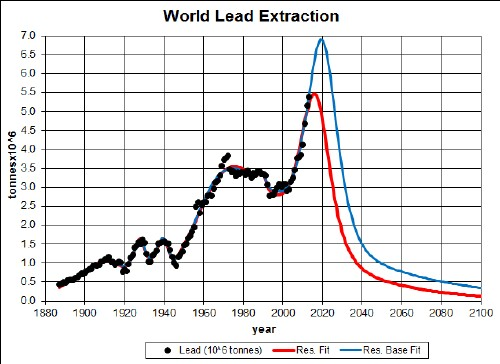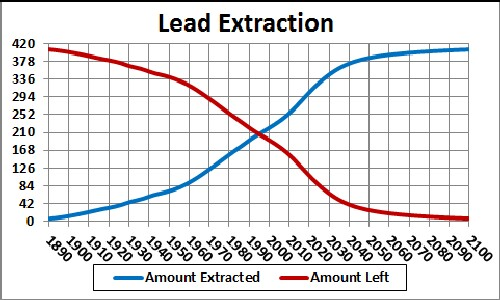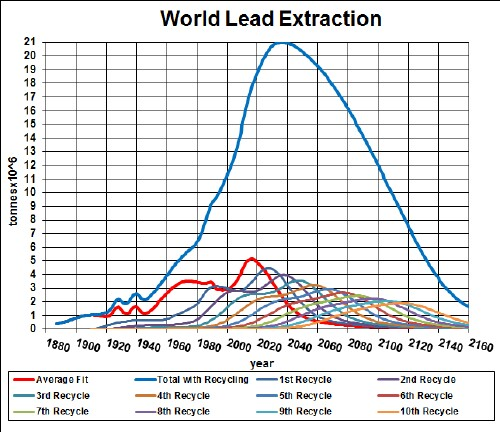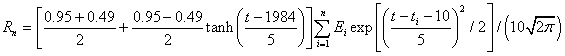Electric vehicles are making a comeback. The low-end electric vehicles use lead-acid batteries. The amount of energy that can be stored in lead-acid batteries is proportional to the amount of lead in the batteries. So, it is important to know about the availability of lead.

The graph below shows the lead extraction data for the world and two Verhulst function fits to the data in order to extrapolate into the future:.Lead extraction rate for the world and two Verhulst functions fits to the data.

The final peak is assumed symmetric; it could be skewed to future times and not be such a high peak.

The red curve is a fit with total amount to be extracted of 340 x 106 tonnes, which incorporates the latest reserves estimate, and the blue curve is fit with total amount to be extracted of 430 x 106 tonnes, which incorporates the latest reserves-base estimate.

Extraction is occurring so rapidly that the depletion curve will peak before 2020.

Taking an average extraction curve of the two fits, the crossover point at year ~1995 when the amount extracted is equal to the amount left to be extracted is shown here:## Recycling

Lead, especially the lead used in batteries, is recycled to a high degree. Assume that:

• The lead-extraction curve is the average of the two curves given above.
• Recycling of lead follows a hyperbolic tangent curve from 30% to 90% recycling with a break point of year 1980 and width 50 years.
• The recycling is delayed by a Gaussian curve peaking at a delay of 20 years and a width of 10 years.

The effective lead available for making items after the first ten recycling cycles is shown in the following graph, along with the effective lead available for each cycle:The equation for a recyling cycle is,

where Ei is the amount available from the previous cycle. Here is an example of the Excel coding:

{=((\$J\$2+\$I\$2)/2+((\$J\$2-\$I\$2)/2)*TANH((A27-\$K\$2)/\$L\$2))*SUM(\$I\$27:I27*(EXP(-1*((A27-\$A\$27:A27-\$N\$2)/\$O\$2)^2/2))/\$O\$2/SQRT(2*PI()))} (The curly bracket surrounding the term makes it into an array; it must be entered by holding down the SHIFT & CTRL keys while pressing the ENTER key.)

Of course, the recycling could be extended to more cycles, skewing the curve further into the future. However, the peak and fall off after it will not change because further cycles are essentally zero in that time region.

Thus, under the assumptions given above, the effective amount of lead available for making items peaks at about 2035 and falls off rapidly after that. Humans will have taken concentrated lead deposits and scattered them across the surface of the earth.

The Excel spreadsheet is set up to make it easy to calculate with different recycling assumptions.